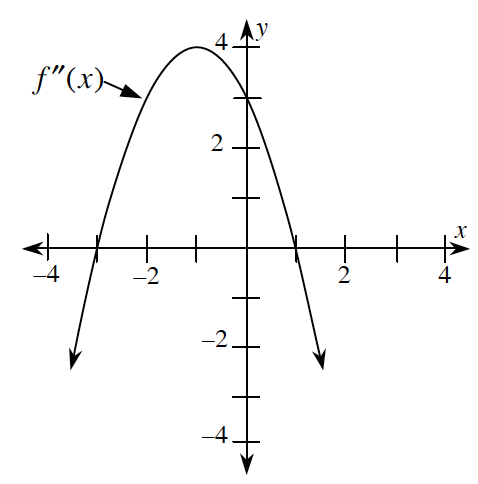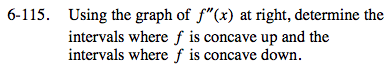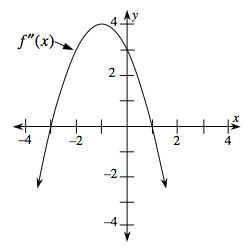### Home > CALC > Chapter 6 > Lesson 6.3.4 > Problem6-115

6-115.

Using the graph of f ″(x) below, determine the intervals where f is concave up and the intervals where f is concave down. Homework Help ✎Notice that you are looking at a graph of y = f ′′(x) but being asked questions about the graph of y = f(x).